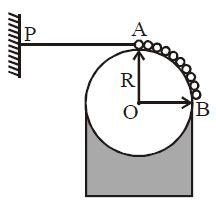# Tension in a Uniform Thread!A uniform flexible chain of mass $m$ and length $L (\leq \frac{\pi R}{2})$ rests on a fixed smooth cylindrical surface of radius $R$ such that one end $A$ of the chain is at the top of the cylinder while the other end $B$ is free. The chain is held stationary by a horizontal thread $PA$ as shown in the figure. Calculate the Tension $(T)$ in the thread.

Assume $\alpha = \dfrac L R$.

×

Problem Loading...

Note Loading...

Set Loading...